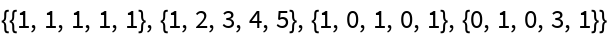#Function Repository Resource:

# SumBasis

Find a basis for the sum of a list of subspaces of ℝ^n

Contributed by: Dennis M Schneider
 ResourceFunction["SumBasis"][list1,list2,…] returns a basis for the sum of the subspaces of ℝn spanned by list1,list2,….

## Examples

### Basic Examples

The sum of a two-dimensional subspace and a three-dimensional subspace of 4:

 In:=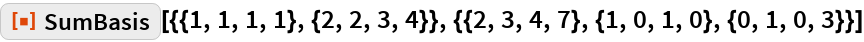Out=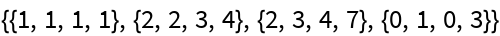The sum of a subspace and a subspace of the subspace:

 In:=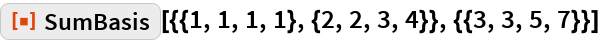Out=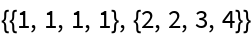A sum of three subspaces:

 In:=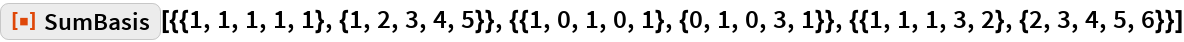Out=